## 6.7 Using Variable Costing to Make Decisions

### Learning Objective

1. Understand how managers use variable costing to make decisions.

In Chapter 2 "How Is Job Costing Used to Track Production Costs?", we discussed how to report manufacturing costs and nonmanufacturing costs following U.S. Generally Accepted Accounting Principles (U.S. GAAP). Under U.S. GAAP, all nonmanufacturing costs (selling and administrative costs) are treated as period costs because they are expensed on the income statement in the period in which they are incurred. All costs associated with production are treated as product costs, including direct materials, direct labor, and fixed and variable manufacturing overhead. These costs are attached to inventory as an asset on the balance sheet until the goods are sold, at which point the costs are transferred to cost of goods sold on the income statement as an expense. This method of accounting is called absorption costingA costing method that includes all manufacturing costs (fixed and variable) in inventory until the goods are sold. because all manufacturing overhead costs (fixed and variable) are absorbed into inventory until the goods are sold. (The term full costing is also used to describe absorption costing.)

Question: Although absorption costing is used for external reporting, managers often prefer to use an alternative costing approach for internal reporting purposes called variable costing. What is variable costing, and how does it compare to absorption costing?

Answer: Variable costingA costing method that includes all variable manufacturing costs in inventory until the goods are sold (just like absorption costing) but reports all fixed manufacturing costs as an expense on the income statement when incurred. requires that all variable production costs be included in inventory, and all fixed production costs (fixed manufacturing overhead) be reported as period costs. Thus all fixed production costs are expensed as incurred.

The only difference between absorption costing and variable costing is in the treatment of fixed manufacturing overhead. Using absorption costing, fixed manufacturing overhead is reported as a product cost. Using variable costing, fixed manufacturing overhead is reported as a period cost. Figure 6.8 "Absorption Costing Versus Variable Costing" summarizes the similarities and differences between absorption costing and variable costing.

Figure 6.8 Absorption Costing Versus Variable Costing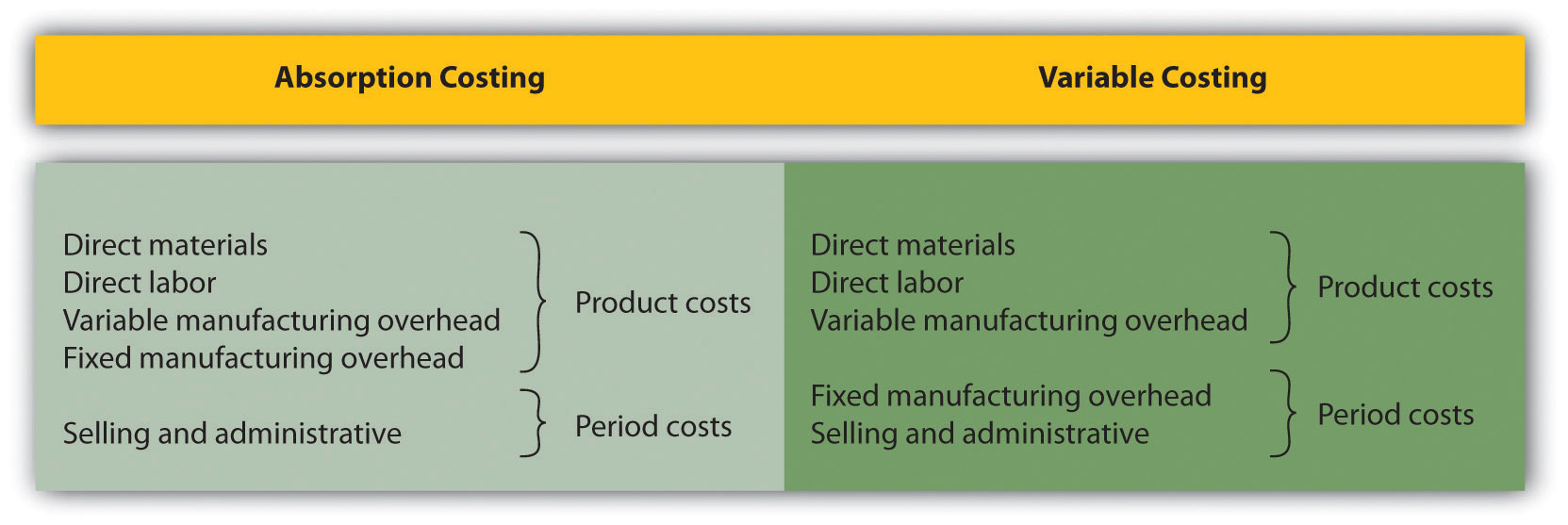## Impact of Absorption Costing and Variable Costing on Profit

Question: If a company uses just-in-time inventory, and therefore has no beginning or ending inventory, profit will be exactly the same regardless of the costing approach used. However, most companies have units of product in inventory at the end of the reporting period. How does the use of absorption costing affect the value of ending inventory?

Answer: Since absorption costing includes fixed manufacturing overhead as a product cost, all products that remain in ending inventory (i.e., are unsold at the end of the period) include a portion of fixed manufacturing overhead costs as an asset on the balance sheet. Since variable costing treats fixed manufacturing overhead costs as period costs, all fixed manufacturing overhead costs are expensed on the income statement when incurred. Thus if the quantity of units produced exceeds the quantity of units sold, absorption costing will result in higher profit.

We illustrate this concept with an example. The following information is for Bullard Company, a producer of clock radios: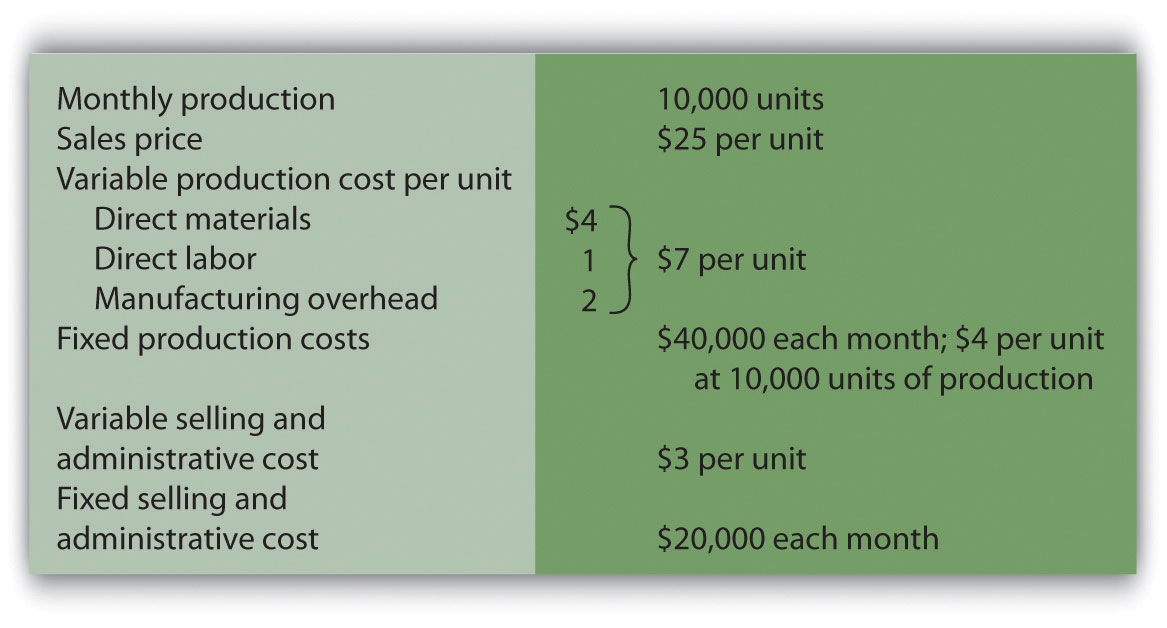Assume Bullard has no finished goods inventory at the beginning of month 1. We will look at absorption costing versus variable costing for three different scenarios:

• Month 1 scenario: 10,000 units produced equals 10,000 units sold
• Month 2 scenario: 10,000 units produced is greater than 9,000 units sold
• Month 3 scenario: 10,000 units produced is less than 11,000 units sold

## Month 1: Number of Units Produced Equals Number of Units Sold

Question: During month 1, Bullard Company sells all 10,000 units produced during the month. How does operating profit compare using absorption costing and variable costing when the number of units produced equals the number of units sold?

Answer: Figure 6.9 "Number of Units Produced Equals Number of Units Sold" presents the results for each costing method. Notice that the absorption costing income statement is called a traditional income statement, and the variable costing income statement is called a contribution margin income statement.

As you review Figure 6.9 "Number of Units Produced Equals Number of Units Sold", notice that when the number of units produced equals the number sold, profit totaling \$90,000 is identical for both costing methods. With absorption costing, fixed manufacturing overhead costs are fully expensed because all units produced are sold (there is no ending inventory). With variable costing, fixed manufacturing overhead costs are treated as period costs and therefore are always expensed in the period incurred. Because all other costs are treated the same regardless of the costing method used, profit is identical when the number of units produced and sold is the same.

Figure 6.9 Number of Units Produced Equals Number of Units Sold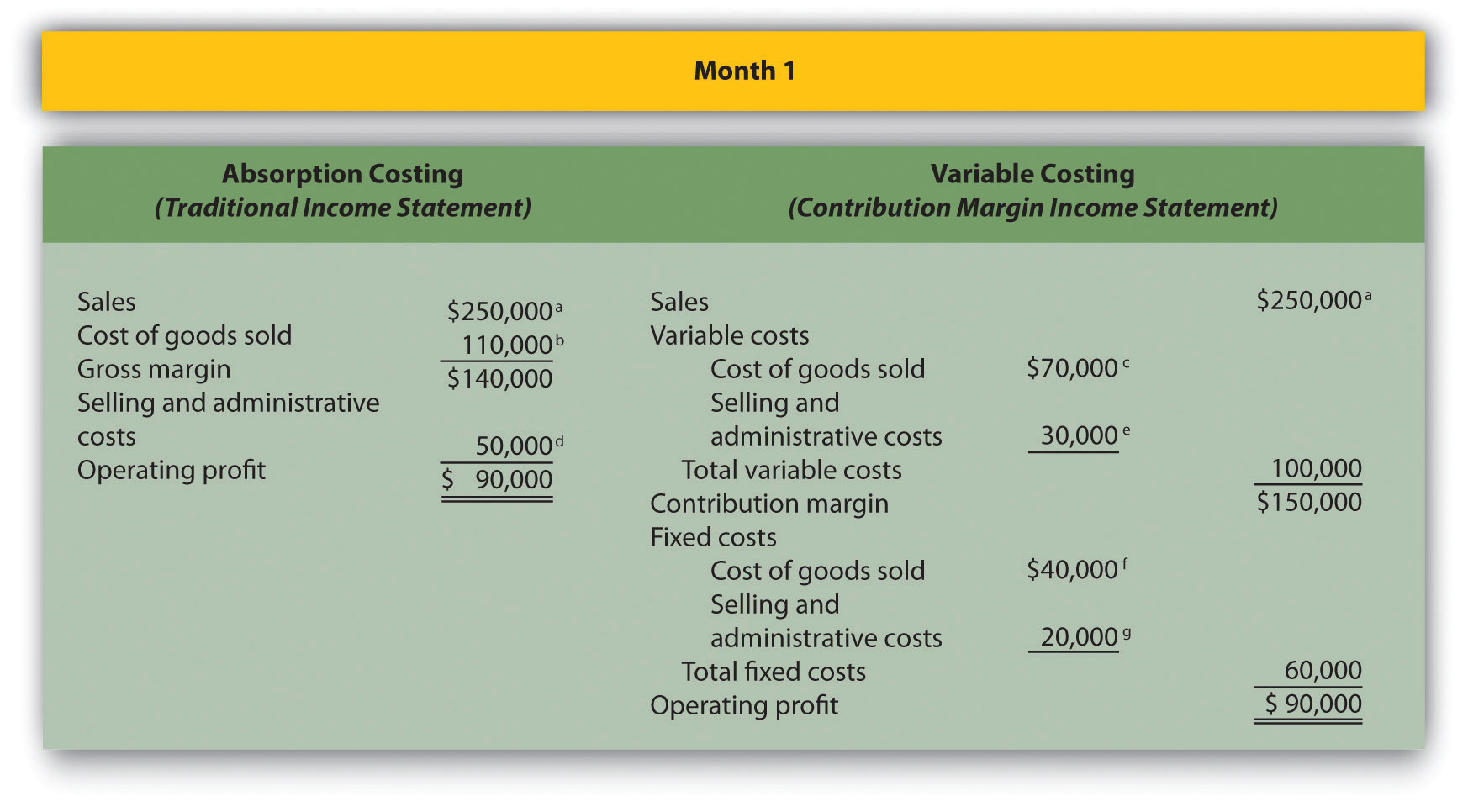a \$250,000 = \$25 × 10,000 units sold.

b \$110,000 = (\$4 per unit fixed production cost × 10,000 units sold) + (\$7 per unit variable production cost × 10,000 units sold).

c \$70,000 = \$7 per unit variable production cost × 10,000 units sold.

d \$50,000 = \$20,000 fixed selling and admin. cost + (\$3 per unit variable selling and admin. cost × 10,000 units sold).

e \$30,000 = \$3 per unit variable selling and admin. cost × 10,000 units sold.

f Variable costing treats fixed manufacturing overhead as a period cost. Thus all fixed manufacturing overhead costs are expensed in the period incurred regardless of the level of sales.

g Given.

## Month 2: Number of Units Produced Is Greater Than Number of Units Sold

Question: During month 2, Bullard Company produces 10,000 units but sells only 9,000 units. How does operating profit compare using absorption costing and variable costing when the number of units produced is greater than the number of units sold?

Answer: Figure 6.10 "Number of Units Produced Is Greater Than Number of Units Sold" presents the results for each costing method. Notice that absorption costing results in higher profit. When absorption costing is used, a portion of fixed manufacturing overhead costs remains in ending inventory as an asset on the balance sheet until the goods are sold. However, variable costing requires that all fixed manufacturing overhead costs be expensed as incurred regardless of the level of sales. Thus when more units are produced than are sold, variable costing results in higher costs and lower profit.

The difference in profit between the two methods of \$4,000 (= \$79,000 − \$75,000) is attributed to the \$4 per unit fixed manufacturing overhead cost assigned to the 1,000 units in ending inventory using absorption costing (\$4,000 = \$4 × 1,000 units).

Figure 6.10 Number of Units Produced Is Greater Than Number of Units Sold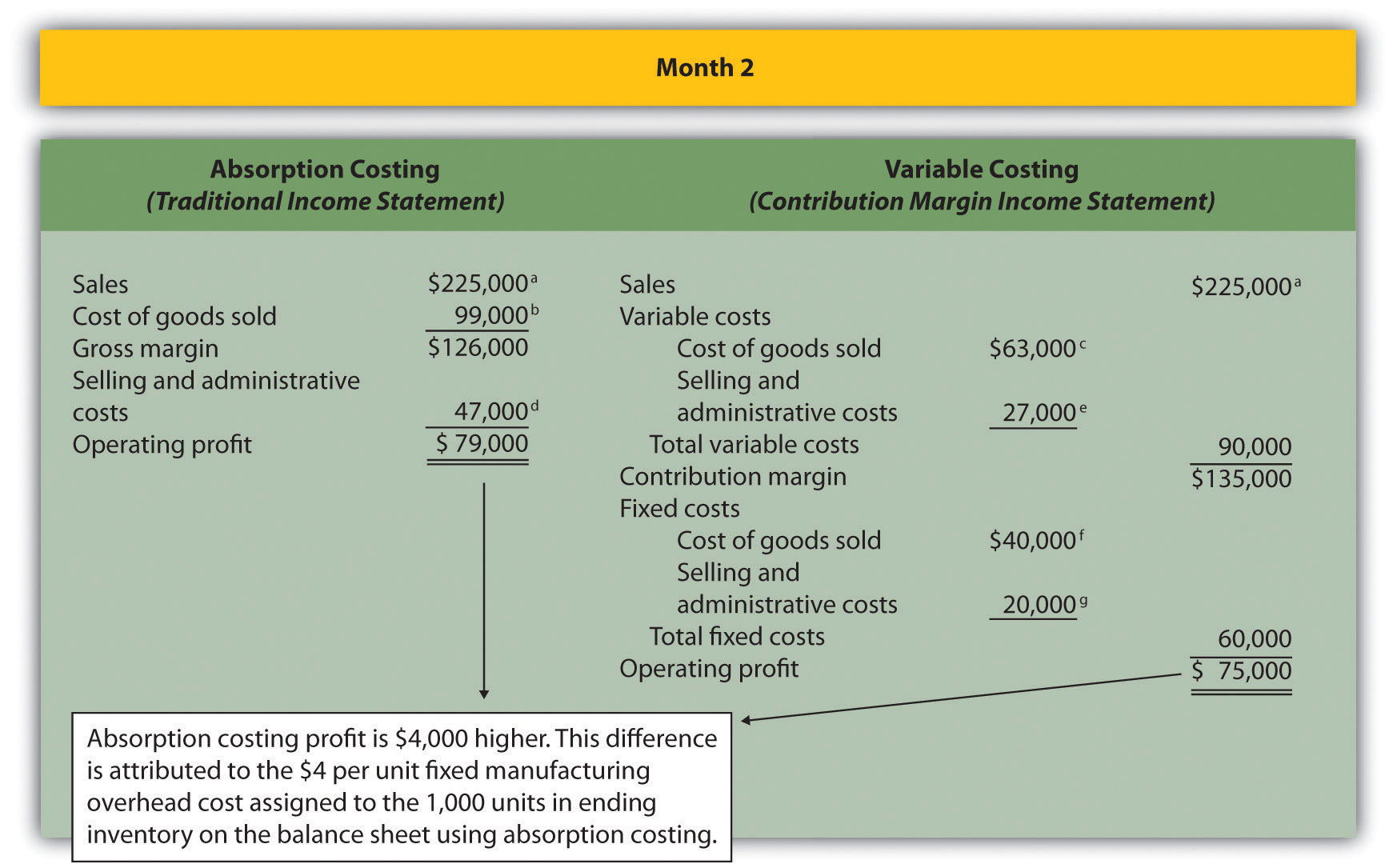a \$225,000 = \$25 × 9,000 units sold.

b \$99,000 = (\$4 per unit fixed production cost × 9,000 units sold) + (\$7 per unit variable production cost × 9,000 units sold).

c \$63,000 = \$7 per unit variable production cost × 9,000 units sold.

d \$47,000 = \$20,000 fixed selling and admin. cost + (\$3 per unit variable selling and admin. cost × 9,000 units sold).

e \$27,000 = \$3 per unit variable selling and admin. cost × 9,000 units sold.

f Variable costing always treats fixed manufacturing overhead as a period cost. Thus all fixed manufacturing overhead costs are expensed in the period incurred regardless of the level of sales.

g Given.

## Month 3: Number of Units Produced Is Less Than Number of Units Sold

Question: During month 3, Bullard Company produces 10,000 units but sells 11,000 units (1,000 units were left over from month 2 and therefore were in inventory at the beginning of month 3). How does operating profit compare using absorption costing and variable costing when the number of units produced is less than the number of units sold?

Answer: Figure 6.11 "Number of Units Produced Is Less Than Number of Units Sold" presents the results for each costing method. Using variable costing, the \$40,000 in fixed manufacturing overhead costs continues to be expensed when incurred. However, using absorption costing, the entire \$40,000 is expensed because all 10,000 units produced were sold; an additional \$4,000 related to the 1,000 units produced last month and pulled from inventory this month is also expensed. Thus when fewer units are produced than are sold, absorption costing results in higher costs and lower profit.

The difference in profit between the two methods of \$4,000 (= \$105,000 − \$101,000) is attributed to the \$4 per unit fixed manufacturing overhead cost assigned to the 1,000 units in inventory on the balance sheet at the end of month 2 and recorded as cost of goods sold during month 3 using absorption costing (\$4,000 = \$4 × 1,000 units).

Figure 6.11 Number of Units Produced Is Less Than Number of Units Sold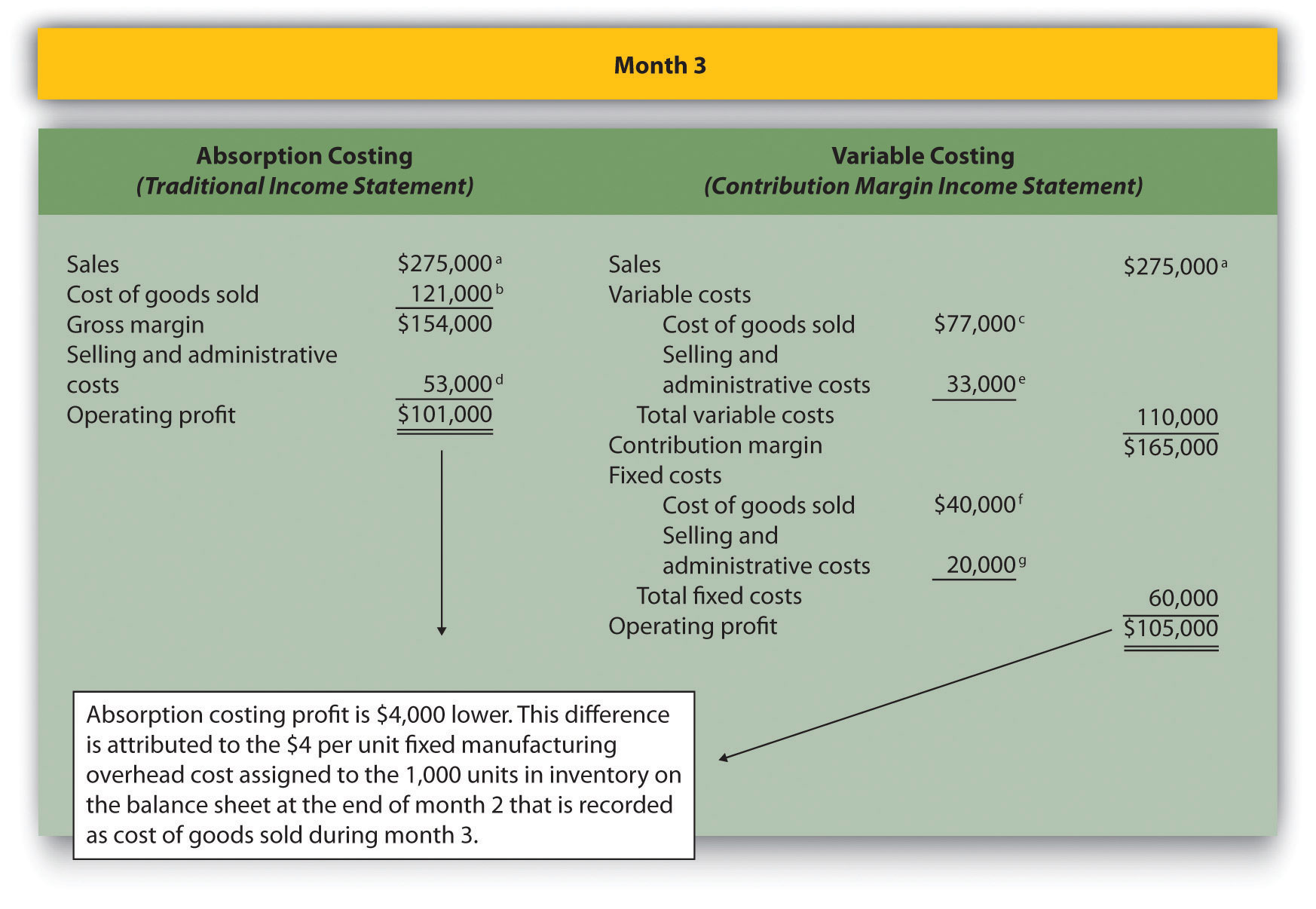a \$275,000 = \$25 × 11,000 units sold.

b \$121,000 = (\$4 per unit fixed production cost × 11,000 units sold) + (\$7 per unit variable production cost × 11,000 units sold).

c \$77,000 = \$7 per unit variable production cost × 11,000 units sold.

d \$53,000 = \$20,000 fixed selling and admin. cost + (\$3 per unit variable selling and admin. cost × 11,000 units sold).

e \$33,000 = \$3 per unit variable selling and admin. cost × 11,000 units sold.

f Variable costing always treats fixed manufacturing overhead as a period cost. Thus all fixed manufacturing overhead costs are expensed in the period incurred regardless of the level of sales.

g Given.

## Advantages of Using Variable Costing

Question: Why do organizations use variable costing?

Answer: Variable costing provides managers with the information necessary to prepare a contribution margin income statement, which leads to more effective cost-volume-profit (CVP) analysis. By separating variable and fixed costs, managers are able to determine contribution margin ratios, break-even points, and target profit points, and to perform sensitivity analysis. Conversely, absorption costing meets the requirements of U.S. GAAP, but is not as useful for internal decision-making purposes.

Another advantage of using variable costing internally is that it prevents managers from increasing production solely for the purpose of inflating profit. For example, assume the manager at Bullard Company will receive a bonus for reaching a certain profit target but expects to be \$15,000 short of the target. The company uses absorption costing, and the manager realizes increasing production (and therefore increasing inventory levels) will increase profit. The manager decides to produce 20,000 units in month 4, even though only 10,000 units will be sold. Half of the \$40,000 in fixed production cost (\$20,000) will be included in inventory at the end of the period, thereby lowering expenses on the income statement and increasing profit by \$20,000. At some point, this will catch up to the manager because the company will have excess or obsolete inventory in future months. However, in the short run, the manager will increase profit by increasing production. This strategy does not work with variable costing because all fixed manufacturing overhead costs are expensed as incurred, regardless of the level of sales.

### Key Takeaway

• As shown in Figure 6.8 "Absorption Costing Versus Variable Costing", the only difference between absorption costing and variable costing is in the treatment of fixed manufacturing overhead costs. Absorption costing treats fixed manufacturing overhead as a product cost (included in inventory on the balance sheet until sold), while variable costing treats fixed manufacturing overhead as a period cost (expensed on the income statement as incurred).

When comparing absorption costing with variable costing, the following three rules apply: (1) When units produced equals units sold, profit is the same for both costing approaches. (2) When units produced is greater than units sold, absorption costing yields the highest profit. (3) When units produced is less than units sold, variable costing yields the highest profit.

### Review Problem 6.8

Winter Sports, Inc., produces snowboards. The company has no finished goods inventory at the beginning of year 1. The following information pertains to Winter Sports, Inc.,: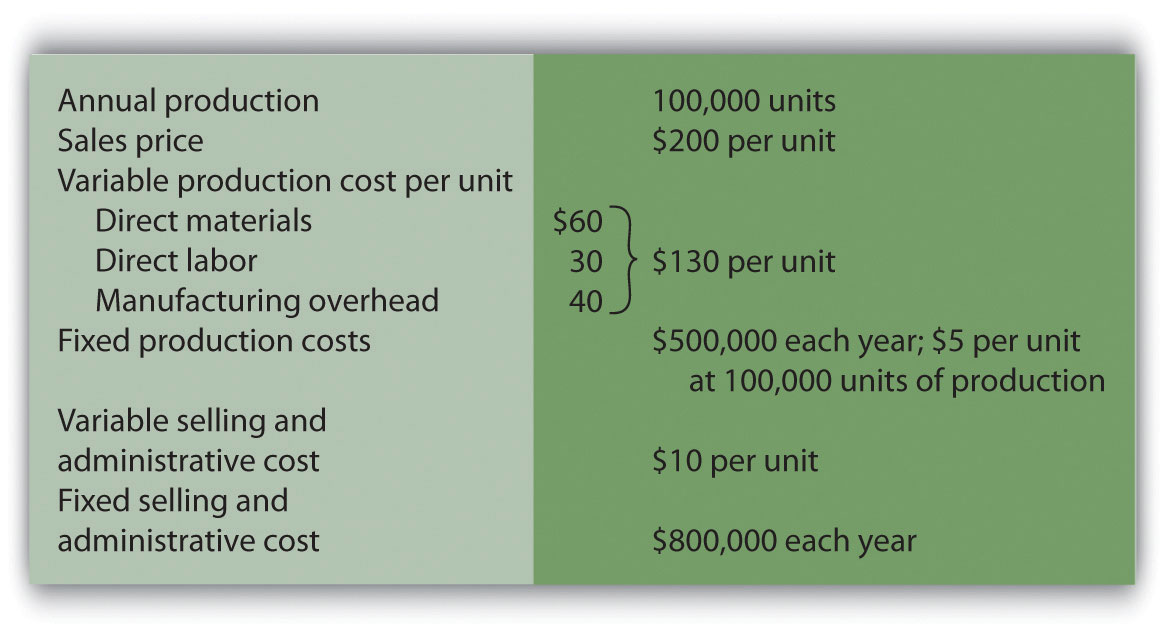1. All 100,000 units produced during year 1 are sold during year 1.

1. Prepare a traditional income statement assuming the company uses absorption costing.
2. Prepare a contribution margin income statement assuming the company uses variable costing.
2. Although 100,000 units are produced during year 2, only 80,000 are sold during the year. The remaining 20,000 units are in finished goods inventory at the end of year 2.

1. Prepare a traditional income statement assuming the company uses absorption costing.
2. Prepare a contribution margin income statement assuming the company uses variable costing.

Solution to Review Problem 6.8

1.

1. Traditional income statement (absorption costing), year 1: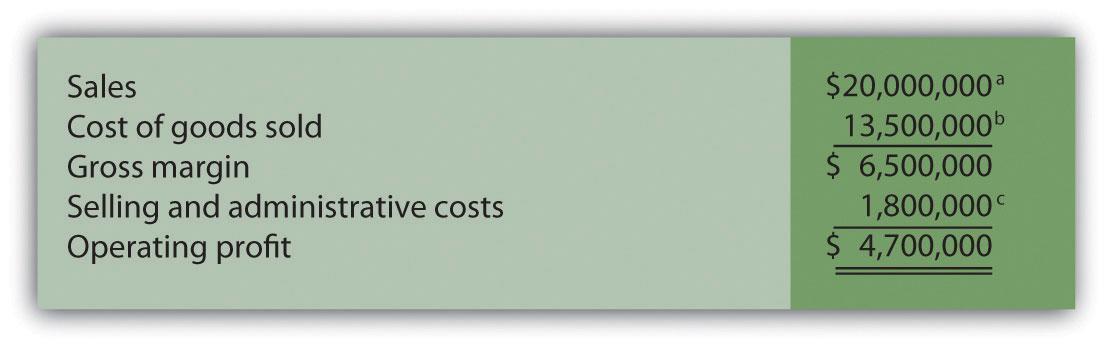a \$20,000,000 = \$200 × 100,000 units sold.

b \$13,500,000 = (\$5 per unit fixed production cost × 100,000 units sold) + (\$130 per unit variable production cost × 100,000 units sold).

c \$1,800,000 = \$800,000 fixed selling and admin. cost + (\$10 per unit variable selling and admin. cost × 100,000 units sold).

2. Contribution margin income statement (variable costing), year 1: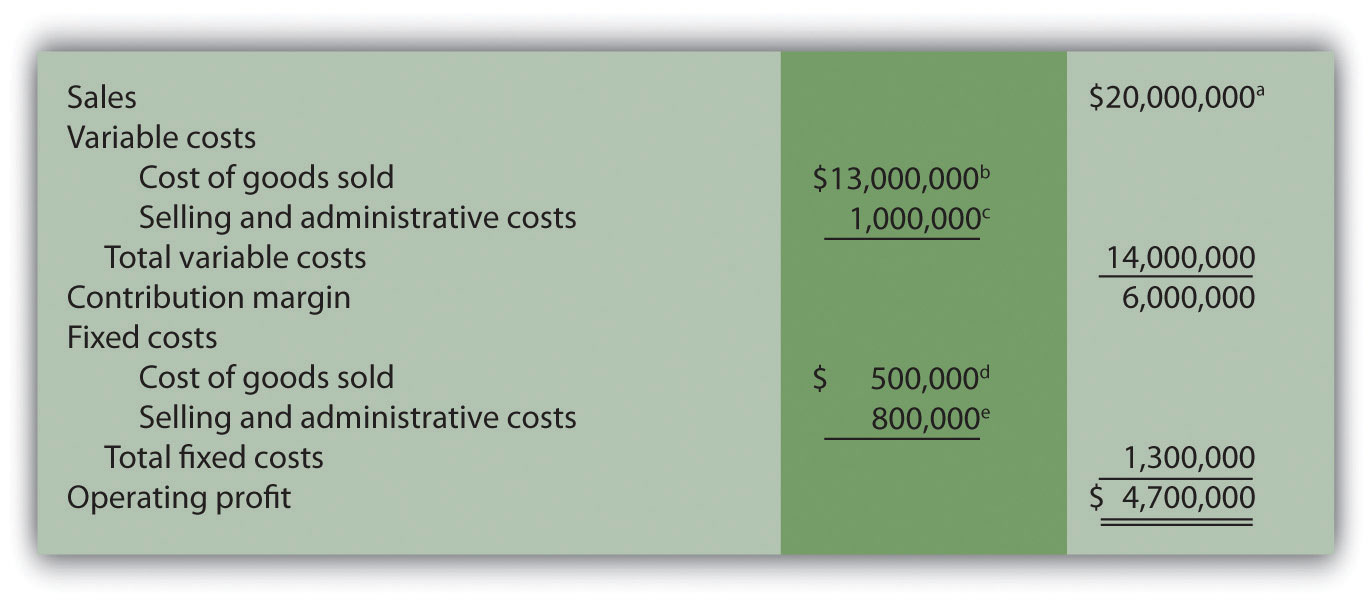a \$20,000,000 = \$200 × 100,000 units sold.

b \$13,000,000 = \$130 per unit variable production cost × 100,000 units sold.

c \$1,000,000 = \$10 per unit variable selling and admin. cost × 100,000 units sold.

d Variable costing treats fixed manufacturing overhead as a period cost. Thus all fixed manufacturing overhead costs are expensed in the period incurred regardless of the level of sales.

e Given.

2.

1. Traditional income statement (absorption costing), year 2: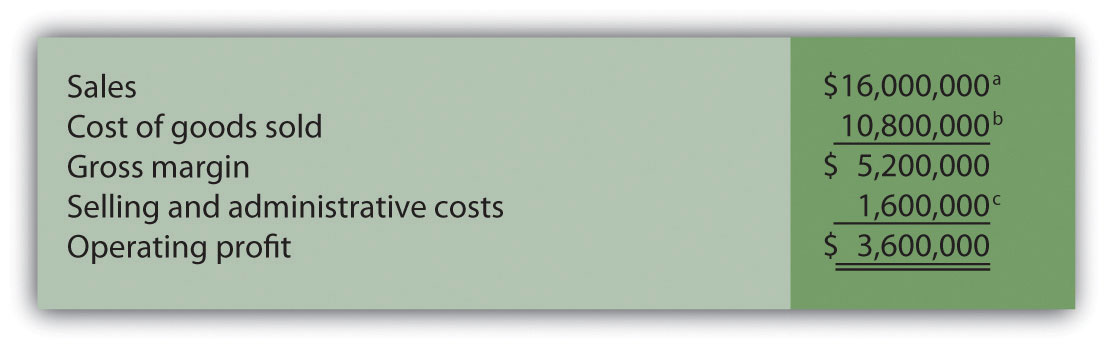a \$16,000,000 = \$200 × 80,000 units sold.

b \$10,800,000 = (\$5 per unit fixed production cost × 80,000 units sold) + (\$130 per unit variable production cost × 80,000 units sold).

2. Contribution margin income statement (variable costing), year 2: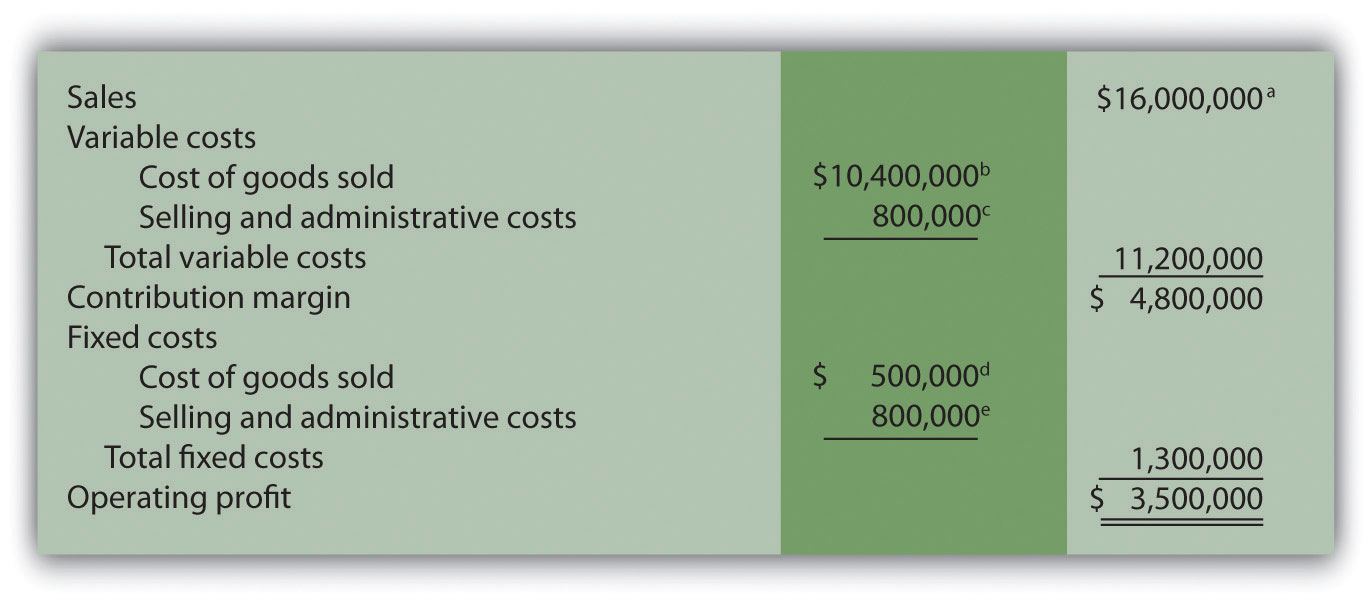a \$16,000,000 = \$200 × 80,000 units sold.

b \$10,400,000 = \$130 per unit variable production cost × 80,000 units sold.

c \$800,000 = \$10 per unit variable selling and admin. cost × 80,000 units sold.

d Variable costing treats fixed manufacturing overhead as a period cost. Thus all fixed manufacturing overhead costs are expensed in the period incurred regardless of the level of sales.

e Given.

### End-of-Chapter Exercises

Questions

1. Describe the components of the profit equation.
2. What is the difference between a variable cost and a fixed cost? Provide examples of each.
3. You are asked to find the break-even point in units and in sales dollars. What does this mean?
4. You are asked to find the target profit in units and in sales dollars. What does this mean?
5. For a company with one product, describe the equation used to calculate the break-even point or target profit in (a) units, and (b) sales dollars.
6. Distinguish between contribution margin per unit and contribution margin ratio.
7. What does the term margin of safety mean? How might management use this information?
8. Review Note 6.16 "Business in Action 6.1" How do airlines measure break-even points? In 2001, which airline had the lowest break-even point?
9. How does the break-even point equation change for a company with multiple products or services compared to a single-product company?
10. Describe the assumptions made to simplify the cost-volume-profit analysis described in the chapter.
11. What is sensitivity analysis and how might it help those performing cost-volume-profit analysis?
12. Review Note 6.37 "Business in Action 6.2" What were the owners concerned about with regards to projected profits? What were the results of the calculations made to address the owners’ concerns?
13. If you are asked to review the cost structure of an organization, what are you being asked to do?
14. When might the contribution margin per unit of constraint be more effective than the contribution margin per unit for making decisions?
15. Describe the three steps used to calculate the target profit for companies that incur income tax costs.
16. Describe the difference between absorption costing and variable costing.
17. Why do some organizations use variable costing?

Brief Exercises

1. Planning at Snowboard Company. Refer to the dialogue at Snowboard Company presented at the beginning of the chapter. What information is Recilia, vice president of sales, requesting from Lisa, the company accountant? How does Recilia plan on using this information?
2. Contribution Margin Calculations. Ace Company sells lawn mowers for \$200 per unit. Variable cost per unit is \$40, and fixed costs total \$4,000. Find (a) the contribution margin per unit, and (b) the contribution margin ratio.
3. Weighted Average Contribution Margin Calculation. Radio Control, Inc., sells radio controlled cars for \$300 per unit representing 80 percent of total sales, and radio controlled boats for \$400 per unit representing 20 percent of total sales. Variable cost per unit is \$150 for cars and \$300 for boats. Find (a) the contribution margin per unit for each product, and (b) the weighted average contribution margin per unit.
4. Sensitivity Analysis, Sales Price. Refer to the base case for Snowboard Company presented in the first column of Figure 6.6 "Sensitivity Analysis for Snowboard Company". Assume the unit sales price decreases by 10 percent. Calculate (a) the new projected profit, (b) the dollar change in profit from the base case, and (c) the percent change in profit from the base case.
5. Sensitivity Analysis, Unit Sales. Refer to the base case for Snowboard Company presented in the first column of Figure 6.6 "Sensitivity Analysis for Snowboard Company". Assume the number of units sold increases by 10 percent. Calculate (a) the new projected profit, (b) the dollar change in profit from the base case, and (c) the percent change in profit from the base case.
6. Operating Leverage. High operating leverage means:

1. The company has relatively low fixed costs.
2. The company has relatively high fixed costs.
3. The company will have to sell more units than a comparable company with low operating leverage to break even.
4. The company will have to sell fewer units than a comparable company with low operating leverage to break even.
5. Both (2) and (3) are correct.
6. Both (1) and (4) are correct.
7. Contribution Margin per Unit of Constraint. Paint Toys Company sells paint ball guns for \$100 per unit. Variable cost is \$60 per unit. Each paint ball gun requires 1.25 machine hours and 2.00 direct labor hours to produce. Calculate the contribution margin (a) per unit, (b) per machine hour, and (c) per direct labor hour.
8. Target Profit with Taxes. Management of Lakewood Company would like to achieve a target profit after taxes of \$300,000. The company’s income tax rate is 40 percent. What target profit before taxes is required to achieve the \$300,000 after-tax profit desired by management?
9. Absorption Costing Versus Variable Costing. Describe the difference between absorption costing and variable costing. Which approach yields the highest profit when the units produced are greater than the units sold? Explain.

Exercises: Set A

1. Break-Even Point and Target Profit Measured in Units (Single Product). Nellie Company has monthly fixed costs totaling \$100,000 and variable costs of \$20 per unit. Each unit of product is sold for \$25.

Required:

1. Calculate the contribution margin per unit.
2. Find the break-even point in units.
3. How many units must be sold to earn a monthly profit of \$40,000?
2. Break-Even Point and Target Profit Measured in Sales Dollars (Single Product). Nellie Company has monthly fixed costs totaling \$100,000 and variable costs of \$20 per unit. Each unit of product is sold for \$25 (these data are the same as the previous exercise):

Required:

1. Calculate the contribution margin ratio.
2. Find the break-even point in sales dollars.
3. What amount of sales dollars is required to earn a monthly profit of \$60,000?
3. Margin of Safety (Single Product). Nellie Company has monthly fixed costs totaling \$100,000 and variable costs of \$20 per unit. Each unit of product is sold for \$25 (these data are the same as the previous exercise). Assume Nellie Company expects to sell 24,000 units of product this coming month.

Required:

1. Find the margin of safety in units.
2. Find the margin of safety in sales dollars.
4. Break-Even Point and Target Profit Measured in Units (Multiple Products). Hi-Tech Incorporated produces two different products with the following monthly data.

 Cell GPS Total Selling price per unit \$100 \$400 Variable cost per unit \$  40 \$240 Expected unit sales 21,000 9,000 30,000 Sales mix 70 percent 30 percent 100 percent Fixed costs \$1,800,000

Assume the sales mix remains the same at all levels of sales.

Required:

1. Calculate the weighted average contribution margin per unit.
2. How many units in total must be sold to break even?
3. How many units of each product must be sold to break even?
4. How many units in total must be sold to earn a monthly profit of \$180,000?
5. How many units of each product must be sold to earn a monthly profit of \$180,000?
5. Break-Even Point and Target Profit Measured in Sales Dollars (Multiple Products). Hi-Tech Incorporated produces two different products with the following monthly data (these data are the same as the previous exercise).

 Cell GPS Total Selling price per unit \$100 \$400 Variable cost per unit \$  40 \$240 Expected unit sales 21,000 9,000 30,000 Sales mix 70 percent 30 percent 100 percent Fixed costs \$1,800,000

Assume the sales mix remains the same at all levels of sales.

Required:

Round your answers to the nearest hundredth of a percent and nearest dollar where appropriate. (An example for percentage calculations is 0.434532 = 0.4345 = 43.45 percent; an example for dollar calculations is \$378.9787 = \$379.)

1. Using the information provided, prepare a contribution margin income statement for the month similar to the one in Figure 6.5 "Income Statement for Amy’s Accounting Service".
2. Calculate the weighted average contribution margin ratio.
3. Find the break-even point in sales dollars.
4. What amount of sales dollars is required to earn a monthly profit of \$540,000?
5. Assume the contribution margin income statement prepared in requirement a is the company’s base case. What is the margin of safety in sales dollars?
6. Changes in Sales Mix. Hi-Tech Incorporated produces two different products with the following monthly data (these data are the same as the previous exercise).

 Cell GPS Total Selling price per unit \$100 \$400 Variable cost per unit \$  40 \$240 Expected unit sales 21,000 9,000 30,000 Sales mix 70 percent 30 percent 100 percent Fixed costs \$1,800,000

Required:

1. If the sales mix shifts to 50 percent Cell and 50 percent GPS, would the break-even point in units increase or decrease? Explain. (Detailed calculations are not necessary but may be helpful in confirming your answer.)
2. Go back to the original projected sales mix. If the sales mix shifts to 80 percent Cell and 20 percent GPS, would the break-even point in units increase or decrease? Explain. (Detailed calculations are not necessary but may be helpful in confirming your answer.)
7. CVP Sensitivity Analysis (Single Product). Bridgeport Company has monthly fixed costs totaling \$200,000 and variable costs of \$40 per unit. Each unit of product is sold for \$50. Bridgeport expects to sell 30,000 units each month (this is the base case).

Required:

For each of the independent situations in requirements b through d, assume that the number of units sold remains at 30,000.

1. Prepare a contribution margin income statement for the base case.
2. Refer to the base case. What would the operating profit be if the unit sales price increases 10 percent?
3. Refer to the base case. What would the operating profit be if the unit variable cost decreases 20 percent?
4. Refer to the base case. What would the operating profit be if total fixed costs decrease 20 percent?
8. CVP Sensitivity Analysis (Multiple Products). Gonzalez Company produces two different products that have the following monthly data (this is the base case).

 Cruiser Racer Total Selling price per unit \$300 \$1,200 Variable cost per unit \$120 \$   720 Expected unit sales 1,400 600 2,000 Sales mix 70 percent 30 percent 100 percent Fixed costs \$180,000

Required:

For each of the independent situations in requirements b through d, assume that total sales remains at 2,000 units.

1. Prepare a contribution margin income statement.
2. Refer to the base case. What would the operating profit be if the Cruiser sales price (1) increases 20 percent, or (2) decreases 20 percent?
3. Refer to the base case. What would the operating profit be if the Cruiser sales volume increases 400 units with a corresponding decrease of 400 units in Racer sales?
4. Refer to the base case. What would the operating profit be if total fixed costs increase five percent? Does this increase in fixed costs result in higher operating leverage or lower operating leverage? Explain.
9. Contribution Margin with Resource Constraints. CyclePath Company produces two different products that have the following price and cost characteristics.

 Bicycle Tricycle Selling price per unit \$200 \$100 Variable cost per unit \$120 \$  50

Management believes that pushing sales of the Bicycle product would maximize company profits because of the high contribution margin per unit for this product. However, only 50,000 labor hours are available each year, and the Bicycle product requires 4 labor hours per unit while the Tricycle model requires 2 labor hours per unit. The company sells everything it produces.

Required:

1. Calculate the contribution margin per unit of constrained resource for each model.
2. Which model would CyclePath prefer to sell to maximize overall company profit? Explain.
10. Target Profit Measured in Units (with Taxes). Optical Incorporated has annual fixed costs totaling \$6,000,000 and variable costs of \$350 per unit. Each unit of product is sold for \$500. Assume a tax rate of 20 percent.

Required:

Use the three steps described in the chapter to determine how many units must be sold to earn an annual profit of \$100,000 after taxes. (Round to the nearest unit.)

11. Target Profit Measured in Sales Dollars (with Taxes). Optical Incorporated has annual fixed costs totaling \$6,000,000 and variable costs of \$350 per unit. Each unit of product is sold for \$500. Assume a tax rate of 20 percent (these data are the same as the previous exercise).

Required:

Use the three steps described in the chapter to determine the sales dollars required to earn an annual profit of \$150,000 after taxes.

12. Absorption Costing Versus Variable Costing. Technic Company produces portable CD players. The company has no finished goods inventory at the beginning of year 1. The following information pertains to Technic Company.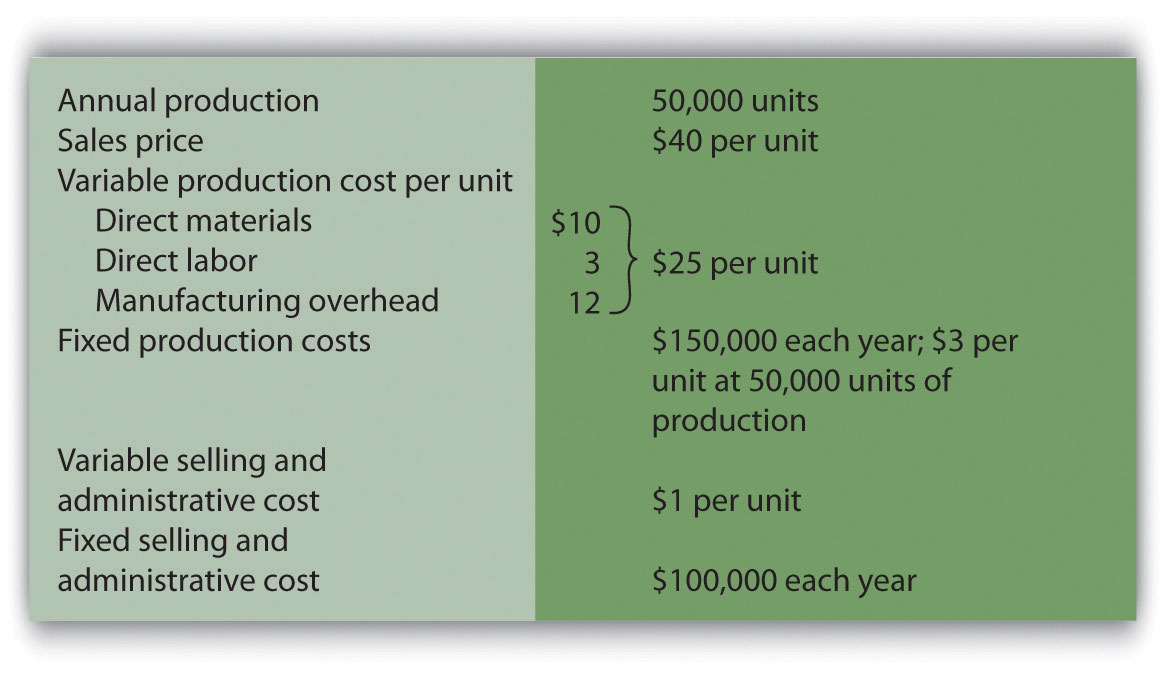Required:

1. All 50,000 units produced during year 1 are sold during year 1.

1. Prepare a traditional income statement assuming the company uses absorption costing.
2. Prepare a contribution margin income statement assuming the company uses variable costing.
2. Although 50,000 units are produced during year 2, only 40,000 are sold during the year. The remaining 10,000 units are in finished goods inventory at the end of year 2.

1. Prepare a traditional income statement assuming the company uses absorption costing.
2. Prepare a contribution margin income statement assuming the company uses variable costing.

Exercises: Set B

1. Break-Even Point and Target Profit Measured in Units (Single Product). Phan Incorporated has annual fixed costs totaling \$6,000,000 and variable costs of \$350 per unit. Each unit of product is sold for \$500.

Required:

1. Calculate the contribution margin per unit.
2. Find the break-even point in units.
3. How many units must be sold to earn an annual profit of \$750,000?
2. Break-Even Point and Target Profit Measured in Sales Dollars (Single Product). Phan Incorporated has annual fixed costs totaling \$6,000,000 and variable costs of \$350 per unit. Each unit of product is sold for \$500 (these data are the same as the previous exercise).

Required:

1. Calculate the contribution margin ratio.
2. Find the break-even point in sales dollars.
3. What amount of sales dollars is required to earn an annual profit of \$300,000?
3. Margin of Safety (Single Product). Phan Incorporated has annual fixed costs totaling \$6,000,000 and variable costs of \$350 per unit. Each unit of product is sold for \$500 (these data are the same as the previous exercise). Assume Phan Incorporated expects to sell 51,000 units of product this coming year.

Required:

1. Find the margin of safety in units.
2. Find the margin of safety in sales dollars.
4. Break-Even Point and Target Profit Measured in Units (Multiple Products). Advanced Products Company produces three different CDs with the following annual data.

 Music Data DVD Total Selling price per unit \$10 \$4 \$12 Variable cost per unit \$  3 \$1 \$  3 Expected unit sales 8,000 10,000 22,000 40,000 Sales mix 20 percent 25 percent 55 percent 100 percent Fixed costs \$205,900

Assume the sales mix remains the same at all levels of sales.

Required:

(Round all answers to the nearest cent and nearest unit where appropriate.)

1. Calculate the weighted average contribution margin per unit.
2. How many units in total must be sold to break even?
3. How many units of each product must be sold to break even?
4. How many units in total must be sold to earn an annual profit of \$200,000?
5. How many units of each product must be sold to earn an annual profit of \$200,000?
5. Break-Even Point and Target Profit Measured in Sales Dollars (Multiple Products). Advanced Products Company produces three different CDs with the following annual data (these data are the same as the previous exercise).

 Music Data DVD Total Selling price per unit \$10 \$4 \$12 Variable cost per unit \$  3 \$1 \$  3 Expected unit sales 8,000 10,000 22,000 40,000 Sales mix 20 percent 25 percent 55 percent 100 percent Fixed costs \$205,900

Assume the sales mix remains the same at all levels of sales.

Required:

Round your answers to the nearest hundredth of a percent and nearest dollar where appropriate. (An example for percentage calculations is 0.434532 = 0.4345 = 43.45 percent; an example for dollar calculations is \$378.9787 = \$379.)

1. Using the information provided, prepare a contribution margin income statement similar to the one in Figure 6.5 "Income Statement for Amy’s Accounting Service".
2. Calculate the weighted average contribution margin ratio.
3. Find the break-even point in sales dollars.
4. What amount of sales dollars is required to earn an annual profit of \$200,000?
5. Assume the contribution margin income statement prepared in requirement a is the company’s base case. What is the margin of safety in sales dollars?
6. Changes in Sales Mix. Advanced Products Company produces three different CDs with the following annual data (these data are the same as the previous exercise).

 Music Data DVD Total Selling price per unit \$10 \$4 \$12 Variable cost per unit \$  3 \$1 \$  3 Expected unit sales 8,000 10,000 22,000 40,000 Sales mix 20 percent 25 percent 55 percent 100 percent Fixed costs \$205,900

Required:

If the sales mix shifts more toward the Data product than the other two products, would the break-even point in units increase or decrease? Explain. (Detail calculations are not necessary, but may be helpful in confirming your answer.)

7. CVP Sensitivity Analysis (Single Product). Skyler Incorporated has monthly fixed costs of \$1,000,000 and variable costs of \$24 per unit. Each unit of product is sold for \$120. Skyler expects to sell 15,000 units each month (this is the base case).

Required:

For each of the independent situations in requirements b through d, assume that the number of units sold remains at 15,000. (Round to the nearest cent where appropriate.)

1. Prepare a contribution margin income statement for the base case.
2. Refer to the base case. What would the operating profit be if the unit sales price decreases 10 percent?
3. Refer to the base case. What would the operating profit be if the unit variable cost increases 10 percent?
4. Refer to the base case. What would the operating profit be if total fixed costs decrease 20 percent?
8. CVP Sensitivity Analysis (Multiple Products). CyclePath Company produces two different products that have the following annual data (this is the base case).

 Bicycle Tricycle Total Selling price per unit \$200 \$100 Variable cost per unit \$120 \$  50 Expected unit sales 5,000 20,000 25,000 Sales mix 20 percent 80 percent 100 percent Fixed costs \$1,000,000

Required:

For each of the independent situations in requirements b through d, assume that total sales remains at 25,000 units.

1. Prepare a contribution margin income statement for the base case.
2. Refer to the base case. What would the operating profit be if the Tricycle sales price (1) increases 10 percent, or (2) decreases 10 percent?
3. Refer to the base case. What would the operating profit be if Bicycle sales volume decreases 500 units and there is a corresponding increase of 500 units in Tricycle sales?
4. Refer to the base case. What would the operating profit be if total fixed costs decrease 10 percent? Does this decrease in fixed costs result in higher operating leverage or lower operating leverage? Explain.
9. Contribution Margin with Resource Constraints. CyclePath Company produces two different products that have the following price and cost characteristics.

 Bicycle Tricycle Selling price per unit \$200 \$100 Variable cost per unit \$120 \$  50

Management believes that pushing sales of the Bicycle product would maximize company profits because of the high contribution margin per unit for this product. However, only 23,000 machine hours are available each year, and the Bicycle product requires 2 machine hours per unit while the Tricycle model requires 1 machine hour per unit. The company sells everything it produces.

Required:

1. Calculate the contribution margin per unit of constrained resource for each model.
2. Which model would CyclePath prefer to sell to maximize overall company profit? Explain.
10. Target Profit Measured in Units (with Taxes). Martis Company has annual fixed costs totaling \$4,000,000 and variable costs of \$300 per unit. Each unit of product is sold for \$400. Assume a tax rate of 20 percent.

Required:

Use the three steps described in the chapter to determine how many units must be sold to earn an annual profit of \$500,000 after taxes. (Round to the nearest unit.)

11. Target Profit Measured in Sales Dollars (with Taxes). Martis Company has annual fixed costs totaling \$4,000,000 and variable costs of \$300 per unit. Each unit of product is sold for \$400. Assume a tax rate of 20 percent (these data are the same as the previous exercise).

Required:

Use the three steps described in the chapter to determine the sales dollars required to earn an annual profit of \$1,000,000 after taxes.

12. Absorption Costing Versus Variable Costing. Photo Company produces digital cameras. The company has no finished goods inventory at the beginning of year 1. The following information pertains to Photo Company.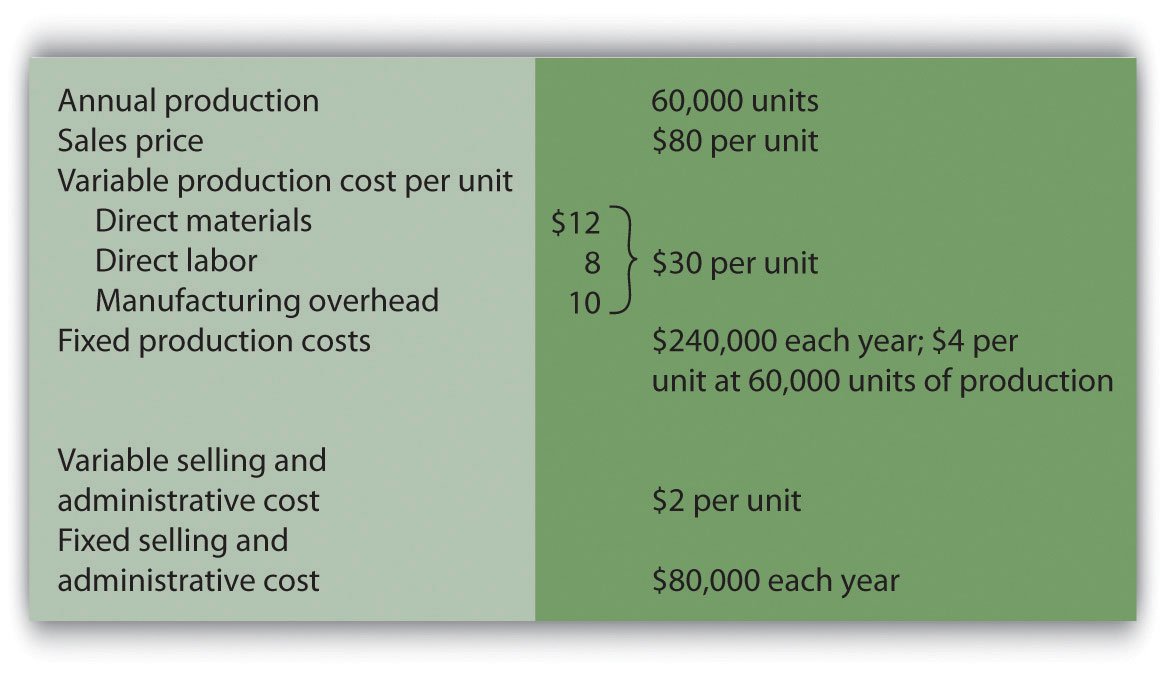Required:

1. All 60,000 units produced during year 1 are sold during year 1.

1. Prepare a traditional income statement assuming the company uses absorption costing.
2. Prepare a contribution margin income statement assuming the company uses variable costing.
2. Although 60,000 units are produced during year 2, only 40,000 are sold during the year. The remaining 20,000 units are in finished goods inventory at the end of year 2.

1. Prepare a traditional income statement assuming the company uses absorption costing.
2. Prepare a contribution margin income statement assuming the company uses variable costing.

Problems

1. CVP and Sensitivity Analysis (Single Product). Madera Company has annual fixed costs totaling \$120,000 and variable costs of \$3 per unit. Each unit of product is sold for \$15. Madera expects to sell 12,000 units this year (this is the base case).

Required:

1. Find the break-even point in units.
2. How many units must be sold to earn an annual profit of \$50,000? (Round to the nearest unit.)
3. Find the break-even point in sales dollars.
4. What amount of sales dollars is required to earn an annual profit of \$70,000?
5. Find the margin of safety in units and in sales dollars.
6. Prepare a contribution margin income statement for the base case.
7. What will the operating profit (loss) be if the sales price decreases 30 percent? (Assume total sales remains at 12,000 units, and round to the nearest cent where appropriate.)
8. Go back to the base case. What will the operating profit (loss) be if the variable cost per unit increases 10 percent? (Assume total sales remains at 12,000 units, and round to the nearest cent where appropriate.)
2. CVP Analysis and Cost Structure (Single Product). Riviera Incorporated produces flat panel televisions. The company has annual fixed costs totaling \$10,000,000 and variable costs of \$600 per unit. Each unit of product is sold for \$1,000. Riviera expects to sell 70,000 units this year.

Required:

1. Find the break-even point in units.
2. How many units must be sold to earn an annual profit of \$2,000,000?
3. Find the break-even point in sales dollars.
4. What amount of sales dollars is required to earn an annual profit of \$500,000?
5. Find the margin of safety in units.
6. Find the margin of safety in sales dollars.
7. How much will operating profit change if fixed costs are 15 percent higher than anticipated? Would this increase in fixed costs result in higher or lower operating leverage? Explain.
3. CVP Analysis with Taxes (Single Product). Riviera Incorporated produces flat panel televisions. The company has annual fixed costs totaling \$10,000,000 and variable costs of \$600 per unit. Each unit of product is sold for \$1,000. Riviera expects to sell 70,000 units this year (this is the same data as the previous problem). Assume a tax rate of 30 percent.

Required:

Round all calculations to the nearest dollar and nearest unit where appropriate.

1. How many units must be sold to earn an annual profit of \$2,000,000 after taxes?
2. What amount of sales dollars is required to earn an annual profit of \$500,000 after taxes?
3. Refer to requirement a. What would happen to the number of units required to earn \$2,000,000 in operating profit if the company were a non-profit organization that did not incur income taxes? Explain. (Detailed calculations are not necessary but may be helpful in confirming your answer.)
4. CVP Analysis and Sales Mix (Multiple Products). Sierra Books Incorporated produces two different products with the following monthly data (this is the base case).

 Text Lecture Notes Total Selling price per unit \$100 \$12 Variable cost per unit \$  60 \$  3 Expected unit sales 21,000 14,000 35,000 Sales mix 60 percent 40 percent 100 percent Fixed costs \$750,000

Assume the sales mix remains the same at all levels of sales except for requirement i.

Required:

Round to the nearest unit of product, hundredth of a percent, and nearest cent where appropriate. (An example for unit calculations is 3,231.15 = 3,231; an example for percentage calculations is 0.434532 = 0.4345 = 43.45 percent; an example for dollar calculations is \$378.9787 = \$378.98.)

1. Calculate the weighted average contribution margin per unit.
2.

1. How many units in total must be sold to break even?
2. How many units of each product must be sold to break even?
3.

1. How many units in total must be sold to earn a monthly profit of \$100,000?
2. How many units of each product must be sold to earn a monthly profit of \$100,000?
4. Using the base case information, prepare a contribution margin income statement for the month similar to the one in Figure 6.5 "Income Statement for Amy’s Accounting Service".
5. Calculate the weighted average contribution margin ratio.
6. Find the break-even point in sales dollars.
7. What amount of sales dollars is required to earn a monthly profit of \$80,000?
8. Assume the contribution margin income statement prepared in requirement d is the company’s base case. What is the margin of safety in sales dollars?
9. If the sales mix shifts more toward the Text product than the Lecture Notes product, would the break-even point in units increase or decrease? Explain. (Detail calculations are not necessary, but may be helpful in confirming your answer.)
5. CVP Analysis and Cost Structure (Service Company). Conway Electrical Services provides services to two types of clients: residential and commercial. The company’s contribution margin income statement for the year is shown (this is the base case). Fixed costs are known in total, but Conway does not allocate fixed costs to each department.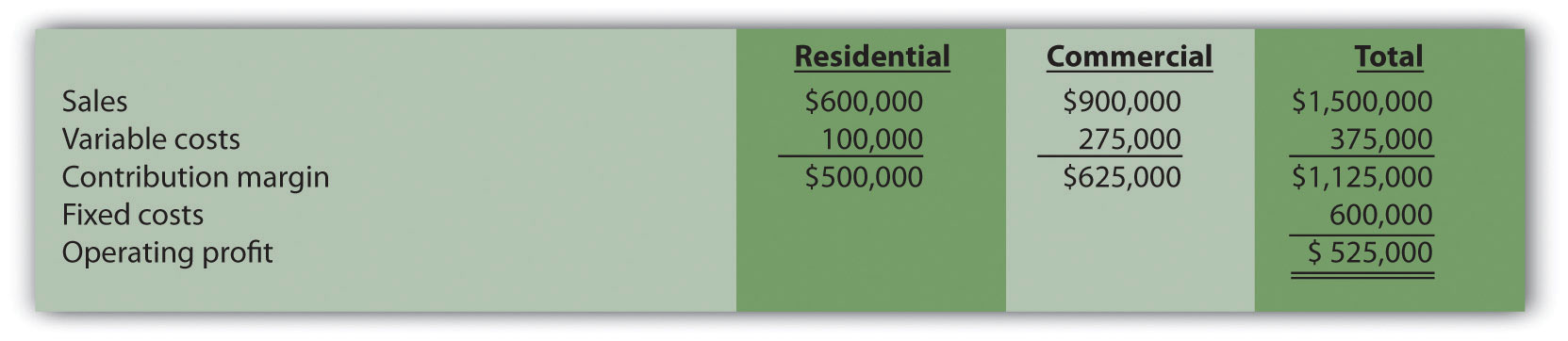Required:

1. Find the break-even point in sales dollars.
2. What is the margin of safety in sales dollars?
3. What amount of sales dollars is required to earn an annual profit of \$750,000?
4. Refer to the base case shown previously. What would the operating profit be if the Commercial variable costs are 20 percent higher than originally anticipated? How does this increase in Commercial variable costs impact the operating leverage of the company?
6. CVP and Sensitivity Analysis, Resource Constraint (Multiple Products). Hobby Shop Incorporated produces three different models with the following annual data (this is the base case).

 Plane Car Boat Total Selling price per unit \$20 \$14 \$24 Variable cost per unit \$  5 \$  7 \$  8 Expected unit sales 30,000 50,000 20,000 100,000 Sales mix 30 percent 50 percent 20 percent 100 percent Fixed costs \$650,000

Assume the sales mix remains the same at all levels of sales except for requirements i and j.

Required:

Round to the nearest unit of product, hundredth of a percent, and nearest cent where appropriate. (An example for unit calculations is 3,231.151 = 3,231; an example for percentage calculations is 0.434532 = 0.4345 = 43.45 percent; an example for dollar calculations is \$378.9787 = \$378.98.)

1. Calculate the weighted average contribution margin per unit.
2.

1. How many units in total must be sold to break even?
2. How many units of each product must be sold to break even?
3.

1. How many units in total must be sold to earn an annual profit of \$500,000?
2. How many units of each product must be sold to earn an annual profit of \$500,000?
4. Using the base case information, prepare a contribution margin income statement for the year similar to the one in Figure 6.5 "Income Statement for Amy’s Accounting Service".
5. Calculate the weighted average contribution margin ratio.
6. Find the break-even point in sales dollars.
7. What amount of sales dollars is required to earn an annual profit of \$400,000?
8. Go back to the base case contribution margin income statement prepared in requirement d. What would the operating profit be if the Plane sales price (1) increases 10 percent, or (2) decreases 10 percent? (Assume total sales remains at 100,000 units.)
9. Go back to the base case contribution margin income statement prepared in requirement d. If the sales mix shifts more toward the Car product than to the other two products, would the break-even point in units increase or decrease? (Detailed calculations are not necessary.) Explain.
10. Assume the company has a limited number of labor hours available in production, and management would like to make efficient use of these labor hours. The Plane product requires 4 labor hours per unit, the Car product requires 3 labor hours per unit, and the Boat product requires 5 hours per unit. The company sells everything it produces. Based on this information, calculate the contribution margin per labor hour for each model (round to the nearest cent), and determine the top two models the company would prefer to sell to maximize overall company profit.
7. Absorption Costing Versus Variable Costing. Wall Tech Company produces wood siding. The company has no finished goods inventory at the beginning of year 1. The following information pertains to Wall Tech Company.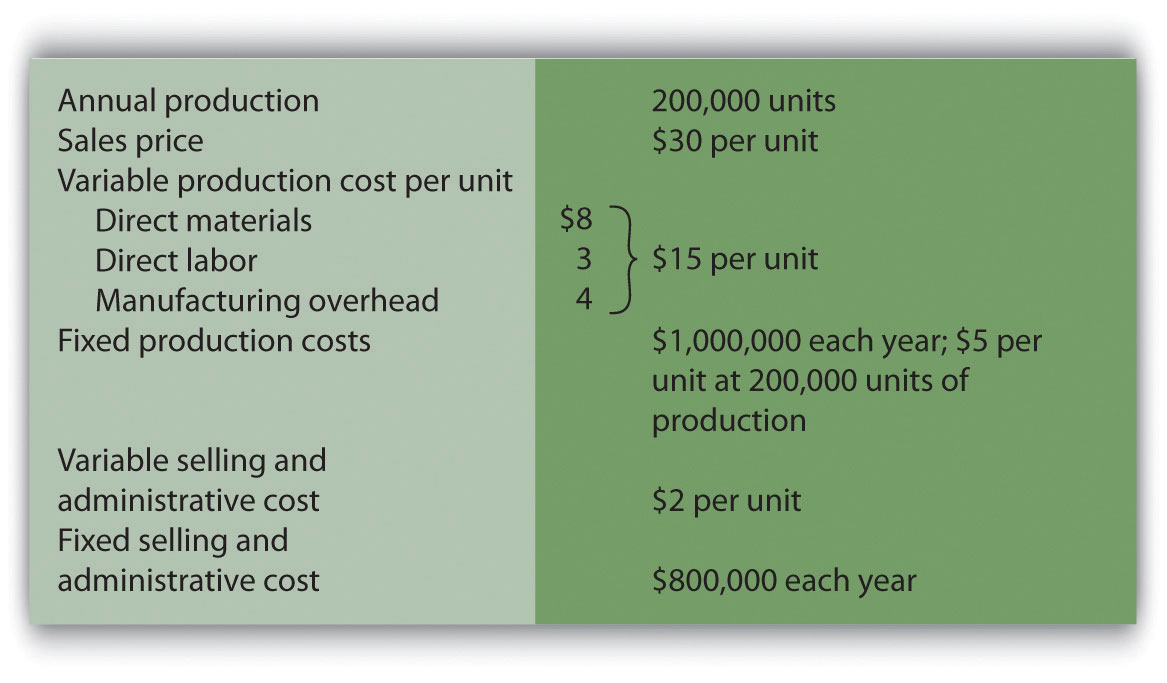Required:

1. All 200,000 units produced during year 1 are sold during year 1.

1. Prepare a traditional income statement assuming the company uses absorption costing.
2. Prepare a contribution margin income statement assuming the company uses variable costing.
2. Although 200,000 units are produced during year 2, only 170,000 units are sold during the year. The remaining 30,000 units are in finished goods inventory at the end of year 2.

1. Prepare a traditional income statement assuming the company uses absorption costing.
2. Prepare a contribution margin income statement assuming the company uses variable costing.
3. Although 200,000 units are produced during year 3, a total of 230,000 units are sold during the year. The 30,000 units remaining in inventory at the end of year 2 are sold during year 3.

1. Prepare a traditional income statement assuming the company uses absorption costing.
2. Prepare a contribution margin income statement assuming the company uses variable costing.
4. Analyze the results in years 1 through 3 (requirements a through c).

One Step Further: Skill-Building Cases

1. Internet Project: CVP Analysis. Using the Internet, go to the Web site for Nordstrom, Inc. (http://www.nordstrom.com), and select investor relations. Find the most recent annual report and print the income statement (called the consolidated statements of earnings).

Required:

1. Calculate the gross profit percentage (also called the gross margin percentage) by dividing the gross profit by net sales.
2. Explain how the gross profit percentage is different than the contribution margin ratio (no calculations are necessary)?
2. Decision Making: Automated Versus Labor Intensive Production. Wood Furniture, Inc., builds high-quality wood desks. Management of the company is considering going from a labor-intensive process of building desks to an automated process that requires expensive machinery and equipment. If the company moves to an automated process, variable production costs will decrease (direct materials, direct labor, and variable manufacturing overhead) due to improved efficiency, and fixed production costs will increase as a result of additional depreciation costs. The costs predicted for the coming year are shown. The selling price is expected to be \$900 per unit for both processes.

 Labor-Intensive Process Automated Process Variable cost of goods sold \$490 per unit \$290 per unit Fixed cost of goods sold (annual) \$1,000,000 \$2,600,000 Variable selling and administrative \$10 per unit \$10 per unit Fixed selling and admin. (annual) \$400,000 \$400,000

Required:

1. Calculate the break-even point in units assuming that (1) the labor-intensive process is used, and (2) the automated process is used.
2. Explain why there is such a significant difference in break-even points between the labor-intensive process and the automated process.
3. Assume Wood Furniture, Inc., expects to produce and sell 8,000 units this coming year and is certain sales will grow by at least 10 percent per year in future years. Calculate the expected operating profit assuming that (1) the labor intensive process is used, and (2) the automated process is used.
4. Using requirement c as a guide, explain whether management should stay with the labor-intensive process or switch to an automated process.
3. Group Activity: Sensitivity Analysis and Decision Making. Performance Sports produces inflatable rafts used for river rafting. Sales have grown slowly over the years, and cost increases are causing Performance Sports to incur losses. Financial data for the most recent year are shown.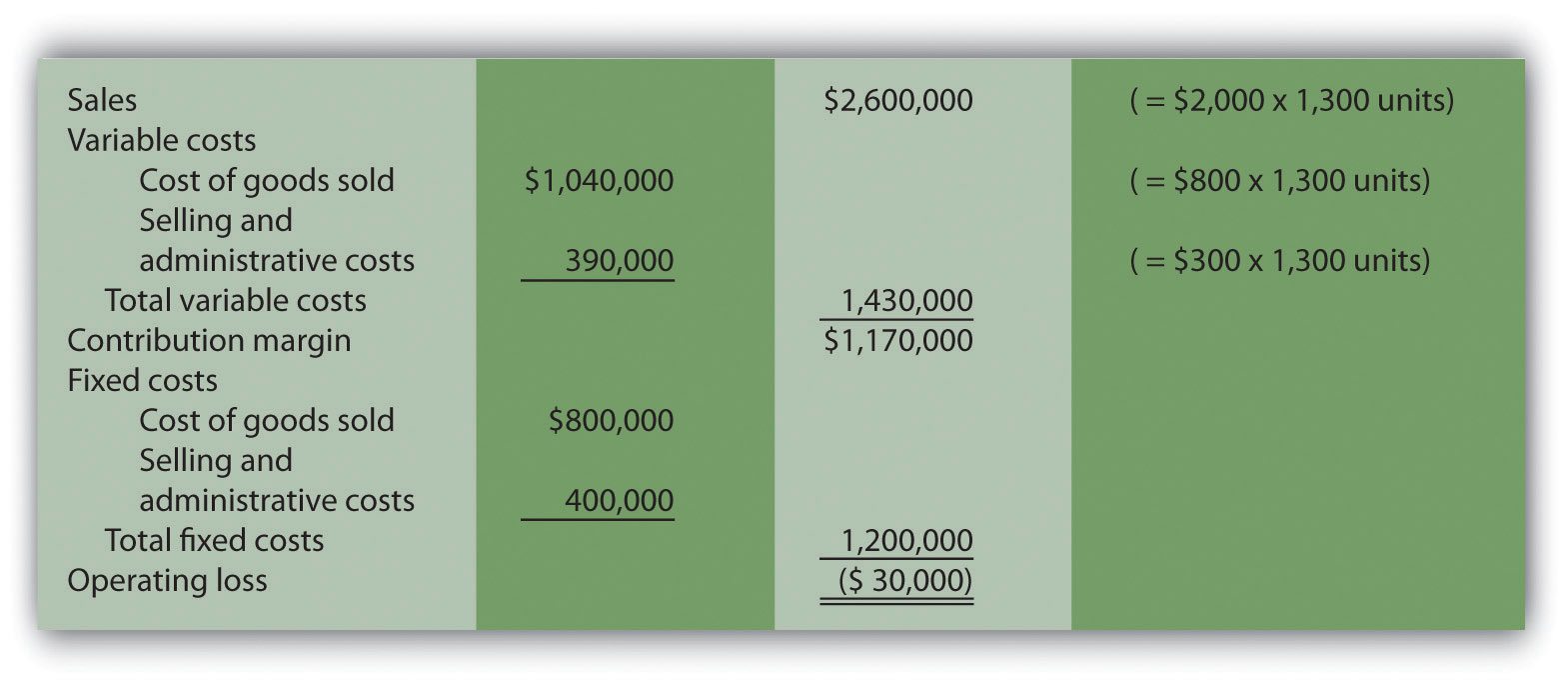Members of the management group at Performance Sports arrived at these three possible courses of action to return the company to profitability (each scenario is independent of the others):

1. Increase the sales price for each raft by 10 percent, which will cause a 5 percent drop in sales volume. Although sales volume will drop 5 percent, the group believes the increased sales price will more than offset the drop in rafts sold.
2. Decrease the sales price for each raft by 10 percent, which will cause an 8 percent increase in sales volume. Although the sales price will drop by 10 percent, the group believes an increase in rafts sold will more than offset the sales price reduction.
3. Increase advertising costs by \$200,000, which will increase sales volume by 15 percent. Although fixed selling and administrative costs will increase by \$200,000, the group believes the increase in rafts sold will more than offset the increase in advertising costs.

Required:

Form groups of two to four students and assign one of the three options listed previously to each group. Each group must perform the following requirements:

1. Calculate the projected operating profit (loss) for the option assigned, and determine whether the option is acceptable.
2. Discuss and document the advantages and disadvantages of the option assigned.
3. As a class, discuss each option based on the findings of your group.
4. Sensitivity Analysis Using Excel. Refer to the information for Performance Sports in Skill-Building Case 60. Prepare an Excel spreadsheet to calculate the operating profit (loss) for the base case and for each of the three scenarios presented in the case. Using the spreadsheet in the Computer Application box in this chapter as a guide, include “data entry” and “sensitivity analysis results” sections, and combine variable cost of goods sold and selling and administrative costs on one line and fixed cost of goods sold and selling and administrative costs on another line.
5. Ethics: Increasing Production to Boost Profit. Hauser Company produces heavy machinery used for snow removal. Over half of the production costs incurred by Hauser are related to fixed manufacturing overhead. Although the company has maximum production capacity of 20,000 units per year, only 2,000 units were produced and sold during year 1, yielding \$25 million in operating losses. As required by U.S. GAAP, the company uses absorption costing.

At the beginning of year 2, the board of directors fired the president of the company and began searching for a new president who was willing to make substantial changes to get the company turned around. One candidate, Paul Glezner, indicated he could turn the company around within a year. He felt the company was producing too few products, and could benefit from increased production. The members of the board of directors were impressed and considered Paul’s contract demands: \$10,000 in base annual salary, plus 30 percent of operating profit. Paul made it clear he would help the company for year 2, but intended to move on after the year ended.

Management of Hauser Company approached you with Paul’s offer and asked you to determine whether the offer is reasonable.

Required:

1. Assume the company’s sales will remain close to 2,000 units in year 2. How does Paul intend to “turn the company around” during year 2?
2. Why do you think Paul insists on leaving the company after year 2?
3. What type of costing system would you recommend Hauser Company use during year 2? Explain.

Comprehensive Case

1. CVP and Sensitivity Analysis for a Brewpub. As described in Note 6.37 "Business in Action 6.2", three entrepreneurs were looking for private investors and financial institutions to fund a new brewpub near Sacramento, California. This brewpub was to be called Roseville Brewing Company (RBC).

Brewpubs provide two products to customers: food from the restaurant segment, and freshly brewed beer from the beer production segment. Both segments are typically in the same building, which allows customers to see the beer brewing process.

After months of research, the three entrepreneurs created a financial model that showed the following projections for the first year of operations: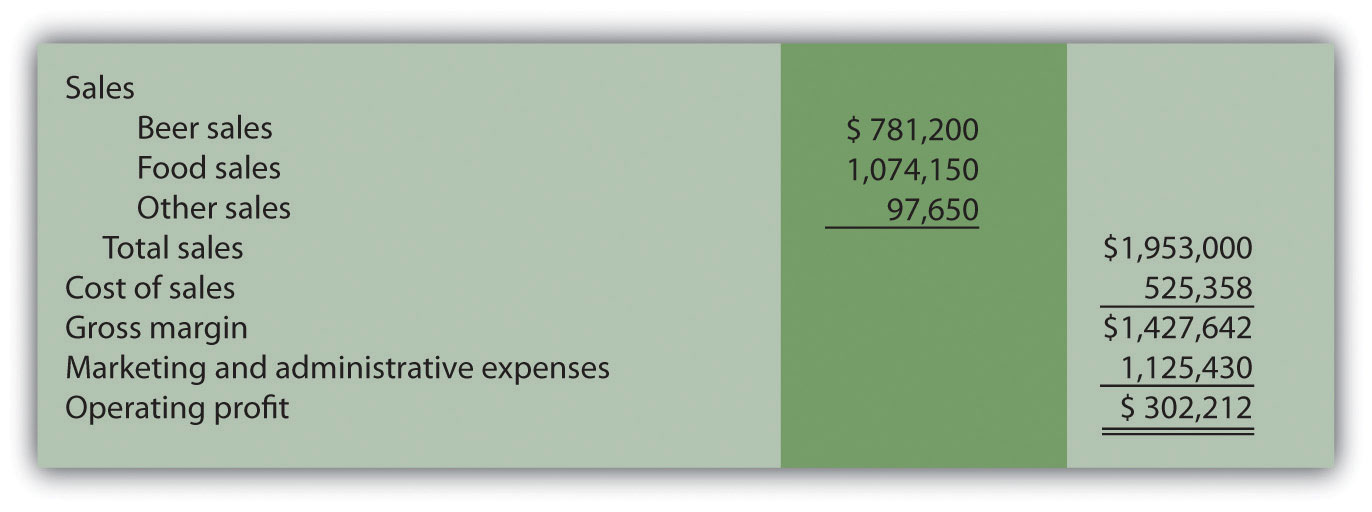In the process of pursuing capital (cash) through private investors and financial institutions, they were asked several questions. The following is a sample of the questions most commonly asked:

• What is the break-even point?
• What sales dollars will be required to make \$200,000? To make \$500,000?
• Is the product mix reasonable? (Beer tends to have a higher contribution margin ratio than food, and therefore product mix assumptions are critical to profit projections.)
• What happens to operating profit if the product mix shifts?
• How will changes in price affect operating profit?
• How much does a pint of beer cost to produce?

It became clear that the initial financial model was not adequate for answering these questions. After further research, the entrepreneurs created another financial model that provided the following information for the first year of operations. (Notice that operating profit of \$302,212 is the same as in the first model.)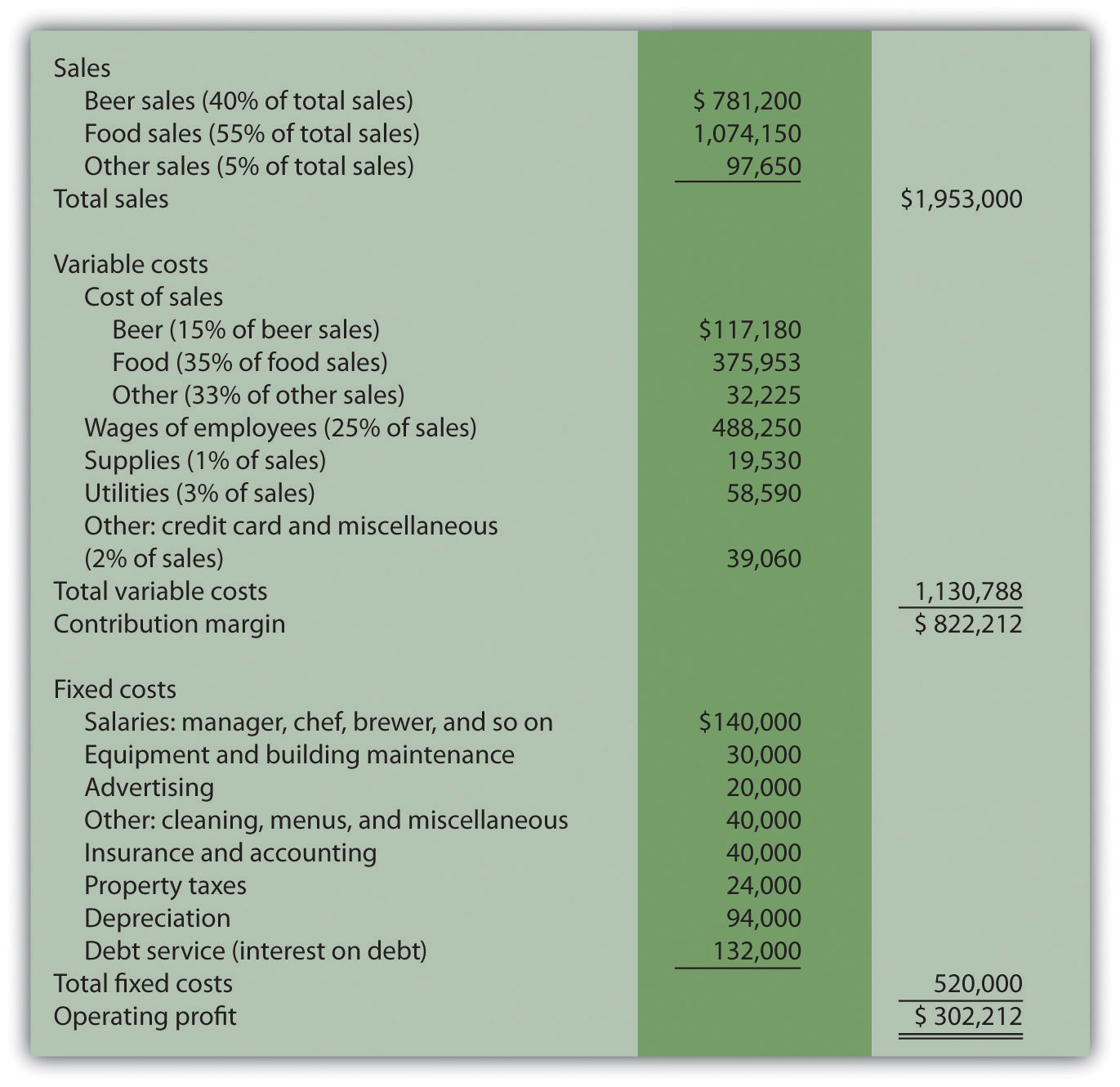Required:

Round your answers to the nearest hundredth of a percent and nearest dollar where appropriate. (An example for percentage calculations is 0.434532 = 0.4345 = 43.45 percent; an example for dollar calculations is \$378.9787 = \$379.)

1. What were potential investors and financial institutions concerned about when asking the questions listed previously?
2. Why was the first financial model inappropriate for answering most of the questions asked by investors and bankers? Be specific.
3. Suppose you are deciding whether to invest in RBC. Which financial ratio would you use to check the reasonableness of RBC’s projected operating profit as compared with that of similar businesses?
4. Why is it difficult to answer the question “How much does a pint of beer cost to produce?” Which costs would you include in answering this question?
5. Perform CVP analysis by answering the following questions:

1. What is the break-even point in sales dollars for RBC?
2. What is the margin of safety in sales dollars for RBC?
3. Why is it not possible for RBC to find the break-even point in units?
4. What sales dollars would be required to achieve an operating profit of \$200,000 and of \$500,000? What assumptions are made in these calculations?
6. Assume total revenue remains the same, but the product mix changes so that each of the three revenue categories is weighted as follows: food 70 percent, beer 25 percent, other 5 percent. Prepare a contribution margin income statement to reflect these changes. How will this shift in product mix affect operating profit?
7. Although the financial model is important, what other strategic factors should RBC and its investors consider?